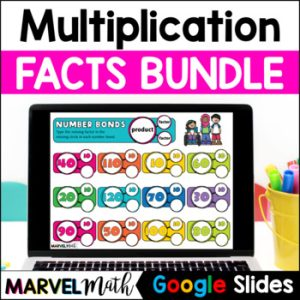Save 10% on your first order with code EXTRA10

# Multiplication Facts x 4 FUN Digital Activities

\$3.00\$19.99
SKU: 5367691 Categories: ,

## Description

The FUN and ENGAGING Multiplication Practice Activities are Perfect for Math Stations, Homework, Tutoring or Distance Learning. Students always need to practice their math facts, and they will enjoy these Simple and Easy-to-Use Google Slides.

This set includes all of the x4 math facts. All Facts 4 x 1 through 4 x 12 and their reverse facts (12 x 4) are included in this resource.

Topics Include:

• Math Facts practice with a mix of movable pieces and places to type in answers to show true memorization
• Missing Factor Equations such as 4 x ? = 20 to help students build the skills needed for division
• Input-Output Tables and Number Machines
• Multiplication Number Bonds

What’s Included?

• PDF with a link to make a copy of the Google Slides in your Google Drive
• 2 Sets of Digital Flashcards for students to use again and again
• 9 Interactive Activities
• Teacher Key
• Directions on how to assign the Google Slides activity to students in Google Classroom

Standards Alignment

CCSS.3.OA.C.7 Fluently multiply and divide within 100, using strategies such as the relationship between multiplication and division (e.g. knowing that 8 x 5 = 40, one knows 40 ÷ 5 = 8) or properties of operations. By the end of Grade 3, know from memory all products of two one-digit numbers.

CCSS.3.OA.A.4 Determine the unknown whole number in a multiplication or division equation relating three whole numbers. For example, determine the unknown number that makes the equation true in each of the equations 8 x ? = 48, 5 = ? ÷ 3, 6 x 6 = ?

TEKS 3.4F Recall facts to multiply up to 10 by 10 with automaticity and recall the corresponding division facts.

TEKS 3.5D Determine the unknown whole number in a multiplication or division equation relating three whole numbers when the unknown is either a missing factor or product.